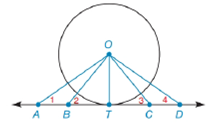Chapter 6.4, Problem 19EElementary Geometry For College St...

7th Edition
Alexander + 2 others
ISBN: 9781337614085

Solutions

Chapter
SectionElementary Geometry For College St...

7th Edition
Alexander + 2 others
ISBN: 9781337614085
Textbook Problem

Given circle O with radius O T ¯ , tangent A D ↔ , and the line segments O A ¯ , O B ¯ , O C ¯ , and O D ¯ :a) Which line segment drawn from O has the shortest length?b) If m ∠ 1 = 40 ° , m ∠ 2 = 50 ° , m ∠ 3 = 45 ° , and m ∠ 4 = 30 ° , which line segment from point O has the greatest length?To determine

(a)

To find:

The line segment drawn from center that has the shortest length.

Explanation

The line that is perpendicular to the radius of a circle at its endpoint on the circle is a tangent to the circle. In the given figure, QT is a tangent to O at point T.

The perpendicular distance is the shortest distance between two points or lines.

Given:

The circle O with radius OT¯, tangent AD, and the line segments OA¯, OB¯

To determine

(b)

To find:

The line segment drawn from center that has the greatest length.

Still sussing out bartleby?

Check out a sample textbook solution.

See a sample solution

The Solution to Your Study Problems

Bartleby provides explanations to thousands of textbook problems written by our experts, many with advanced degrees!

Get Started

In Exercises 4562, find the values of x that satisfy the inequality (inequalities). 59. x+3x20

Applied Calculus for the Managerial, Life, and Social Sciences: A Brief Approach

Find the value of the sum. 30. i=1n(25i)

Single Variable Calculus: Early Transcendentals, Volume I

Construct a drawing of the fourth hexagonal number.

Mathematical Excursions (MindTap Course List)

Graphing Inequalities 12. x 1

Precalculus: Mathematics for Calculus (Standalone Book)

22. Let . Prove .

Elements Of Modern Algebra

01e2xdx= a) 2e2 1 b) `e2 1 c) 12e2 d) 12(e21)

Study Guide for Stewart's Single Variable Calculus: Early Transcendentals, 8th